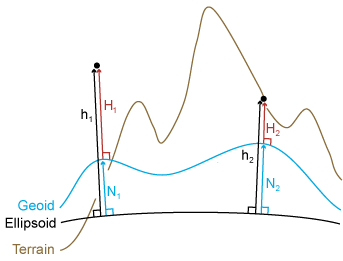## Find Ellipsoidal Height from Orthometric Height

The height of an object may refer to its ellipsoidal height or its orthometric height. Mapping Toolbox™ functions such as `geodetic2enu` require the input argument ellipsoidal height, but data often quantifies orthometric height instead. You can convert orthometric height to ellipsoidal height by using a geoid model.

Ellipsoidal height, called h, is height measured along a normal of a reference ellipsoid. For more information about reference ellipsoids, see Reference Spheroids. This image shows a positive ellipsoidal height, hp, and a negative ellipsoidal height, hn.Most terrain models provide data using orthometric height instead of ellipsoidal height. Orthometric height, called H, is height above the geoid.

The geoid models the average sea level of the Earth without effects such as weather, tides, and land. A geoid model is created by measuring variations in the Earth's gravitational field, so it has a smoothly undulating shape. Orthometric height is measured relative to the geoid.

Geoid height, called N, is the height of the geoid measured along a normal of a reference ellipsoid. For example, geoid height values from the Earth Gravitational Model of 1996 (EGM96) are referenced to the ellipsoid defined by the World Geodetic System of 1984 (WGS84). Below is an illustration of the geoid from EGM96, with geoid heights in meters.To find ellipsoidal height at a specified latitude and longitude, add the orthometric height and geoid height: h = H + N. You can find the height of the geoid from EGM96 at specified latitudes and longitudes using the `egm96geoid` function.

The equation h = H + N is an approximation because the direction along which a geoid height is measured is not necessarily the same as the direction along which an orthometric height is measured. However, the approximation is suitable for most practical purposes.

The following image illustrates the relationship between ellipsoidal height, orthometric height, and the geoid. The values h1, H1, and N1 demonstrate the relationship for an airborne object, while h2, H2, and N2 demonstrate the relationship for an object on land.### Find Ellipsoidal Height from Orthometric and Geoid Height

Find the ellipsoidal height of the summit of Mount Everest, using its orthometric height and a geoid model.

Specify the latitude and longitude of the summit in degrees. Specify the orthometric height in meters.

```lat = 27.988056; lon = 86.925278; H = 8848;```

Find the height of the geoid at the location specified by `lat` and `lon` using `egm96geoid`.

`N = egm96geoid(lat,lon);`

Calculate the ellipsoidal height of the summit.

`h = H + N`
```h = 8.8193e+03 ```

 NOAA. "What is the geoid?" National Ocean Service website. https://oceanservice.noaa.gov/facts/geoid.html, 06/25/18.